CalGraph Bob's Calculus## The Cauchy-Schwartz Inequality

What is it?

The Cauchy-Schwartz  Inequality  |<U,W>|      ||U||∙ ||W||      is an example of a lemma.

"Lemma" is Latin for "unimportant theorem".

( Not exactly:  a lemma is a result which is needed to prove a more general or harder theorem .)

Where did it come from?

We can visualize it and explore its origin at the same time.

Let's start with the assumption that the shortest distance between two points on a flat (See Note 1) surface is a straight line.

In the diagram, it's a shorter walk from O to B, than from O to A and then from A to B.(By the way, the diagram was generated using Calgraph, a Graphing/Statistics/Calculus Calculator created by Kishore Anand. You can download it at http://math-interactive.com/download.html and run it on any Windows laptop or desktop.)

Let's call the vector joining O to A:  U = (u1  , u2).

Similarly, after reaching the point A, we walk parallel to the vector  W = (w1 , w2).                             (Line 1)

If we walked directly from  O to  B, we would be at the point with coordinates:  (u1 + w1 , u2 + w2).

So, translating our statement about the shortest distance between two points being a straight line into vector algebra, ||U + W|| ||U|| + ||W||

Since all the quantities are non-negative, the preceding inequality is still true if we square it. (The reason to square it is to avoid having to deal with square roots.)

|| U + W ||2   ( ||U|| + ||W||)2

||(u1 + w1 , u2 + w2)||2  ||U||2+ ||W||2  +  2||U|| ∙||W||

(u1 + w1)2+( u2 + w2)2  ||( u1 ,  u2)||2+|| (w1  , w2)||2 + 2 ||U||∙ ||W||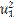+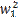+ 2 u1 w1  +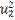+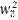+2 u2 w2   ||( u1 , u2)||2+|| (w1  , w2)||2 + 2||U||∙ ||W||

Rearranging the terms on the left side:

(+) + (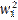+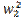) + 2 u1 w1 + 2 u2 w2   ||( u1 , u2)||2+|| (w1 , w2)||2 +2 ||U||∙ ||W||

On the left,  the terms in parentheses  are  exactly ||( u1 , u2)||2 and ||(w1 , w2)||2 respectively. Subtracting them from both sides and then dividing everything by 2 we have:

u1 w1+ u2 w2    ||U|| ∙||W||

But the left is exactly the dot or  inner product < U, W > of the two vectors  U and W , so we have:

|< U, W >|    ||U|| ∙||W||                                                                                                                  (Line 2)

(If you are unsatisfied, see Note 2.)

Who needs it?

The following  three applications can be proved in one of two ways:  directly or by showing that the relations  live in a normed vector space, so that they derive from the general Cauchy-Schwartz Inequality.   However, either type of proof demands a knowledge of subjects traditionally taught after Vector Algebra. (Quantum Mechanics,  Integration and Infinite Series).

1   Physics: Arguably, the most important tenet of Quantum Mechanics is a direct consequence of the Cauchy-Schwartz Inequality:

The Heisenberg Uncertainty Principle: There is a fundamental limit to the precision to which certain pairs of physical quantities of a particle can simultaneously be measured.

e.g.  A particle's position x and momentum p: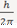∆x ∙∆p

For example, the more accurately the position is measured (∆x small), the greater will be the uncertainty in the momentum (∆p), as the product of the two errors must be at least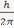.

An elegant, fairly simple proof on the Uncertainty Principle appears here:

http://www.phys.ufl.edu/courses/phy4604/fall18/uncertaintyproof.pdf

2  If f and g are integrable functiona over domain E: (f* is the complex conjugate of f)

|∫E  f*g dx|      ( E  | f |2 dx)1/2 ( E  |g|2 dx)1/2

3  (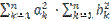Taking the limits of both sides as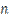→∞, this inequality can be used in convergence proofs.

(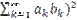Here's the skeleton of a proof by Mathematical Induction:

(The n=1 case is trivial and the Algebra for the n=2 case is instructive for the Induction Step.)

We will need: 0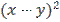=+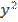- 2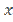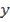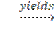2+(Line 3)

(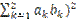==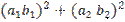+2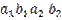+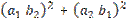= (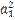+(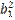+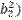=(The inequality comes from replacing x by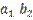and y by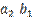in  (Line 3).

The Induction Step can be accomplished with analogous algebra to the last few lines.

How do you know it's valid?

The general proof is constructive, in other words,  a cute trick.

U and W are vectors in an Inner Product Space over a field F.    (See Note 3)

Consider  U- α W (where α ε  F)

We will assume that W 0 as that case is trivial.

(Line 4)

0 ≤   < U- α W, U- α W >     (true for the inner product of any vector with itself, equality only for 0)

Expanding the left hand side:

<U, U- α W > -  α <W, U- α W > = <U, U> - α *<U, W> - α <W, U> + α∙ α* <W, W>

Set  α  =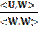(No worries about a 0 denominator as W was assumed to be a non-zero vector.)

0      < U- α W, U- α W >  =

<U, U><U, W> -<W, U> +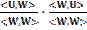<W, W>                                       (Line 4)

Simplifying the preceding line:

0        <U, U> -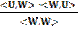A little Algebra and the realization that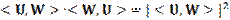and  <U, U> =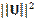yields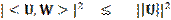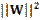or simply:  |<U, W>|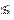||U||||W||                                                                                           ( Line 5)

Incidentally, tracing back to close to the beginning of the proof  ( Line 4), we have equality if and only if  U = α W,  so equality occurs only when  U and W are linear multiples.

i.e. Inor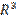equality occurs in the Cauchy-Schwartz Inequality (Line 5) only if U and W are parallel vectors.

Notes:

1) By normal, flat 2-dimensional space, we mean the surface of a piece of paper as opposed to the curved 2-dimensional surface of, for example,  a sphere or a bagel.

In real two dimensional real space, the inner product is the same as the dot product of two vectors.

So if U = (u1  , u2) and W = (w1 , w2)

<U, W> = U  W = (u1  , u2)  ∙ (w1 , w2)  = 2 u1 w1  + 2 u2 w2

2) The eagle-eyed reader might have noticed a hole in the proof.  The absolute value in Line 2 wasn't justified. However, if, after Line 1,  point B had been taken as (u1 -  w1 , u2 -  w2), then  proceeding analogously,  the left side of Inequality I would have had been multiplied by -1 . Thus,  the absolute value at Line 2  can be justified.

3) Definition of an Inner Product Space.

An inner product space is a vector space V over a field F (F = R or C) together with an operation <. , .>

<. , .>: VxV -> F

(The idea is that the inner product is a function with two vectors as the input and a single real or complex number as the output.)

·         Conjugate symmetry:

<U, W> = <U, W>C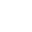·        Linearity in the first argument:

< αU,w> = α <U,W>

<U + V,W> = <U,W> + <V,W>·        Positive Definite:  <U,U>   0   with equality only if U  is the 0 vector.

4) Sometimes, Mathematicians develop abstract systems for their own amusement and are surprised or even miffed when their work proves useful. For example, Group Theory was unknown outside pure Mathematics from its inception circa 1855 until 1920 when Weyl rolled it into Quantum Mechanics. Inner product spaces are generalizations of our familiar R2 and, as one can see from the applications of just this Inequality, have proved to be extremely useful.